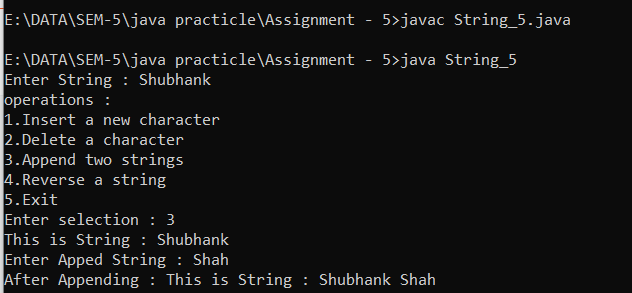## Insert A New Character | Delete A Character | Append Two Strings | Reverse A String

Output Code import java.util.Scanner; abstract class abs{ StringBuffer str; Scanner scan=new Scanner(System.in); abstract void ins(); ...

## Find A Substring | Find A Length of The String | Find A Particular Character Located At Entered Index Number

Output Code import java.util.Scanner; interface st{ void Sub(); void len(); void index(); } class menu implements st{ Scanner scan...

## Write Down The Equation And Transformation Matrix For 3D Rotation About X, Y and Z-Axis

• For 3-D rotation, we need to pick an axis to rotate an object. • The most common choices are the x-axis, y-axis, and z-axis. X-Axis Rota...

## Derive The Equation And Transformation Matrix For Producing Parallel Projection On 2D Viewing Plane And Also Explain Orthographic And Oblique Projections

• Parallel projection methods are used by engineers to create and working drawing of an object which preserves its true value. • In the case...

## Given a Clipping Window P(20,20), Q(60,20), R(60,40) and S(20,40), Use Cohen Sutherland To Determine The Visible Portion of The Line A(40,80) and B(120,30)

Solution Clipline (40, 80, 120, 30, 20, 60, 20, 40) { code1 = 0000  done = 0 code2 = 0000 draw = 0 while (done == 0) { code1 ...

## Double Buffering In Computer Graphics

Double Buffering Image Source - Google | Image By - slideshare • The idea of maintaining 2 images, one to show and one to build or alter i...

## Segments In Computer Graphics

Segments Image Source - Google | Image By -  geeksforgeeks • The segment name is zero then it is called an undefined segment. • BD created...

## Liang Barsky Line Clipping Algorithm In Computer Graphics

Liang Barsky Algorithm Image Source - Google | Image By -  pracspedia • Parametric equation of line is given by, x = x1 + (x2 - x1) * u y = ...

### Insert A New Character | Delete A Character | Append Two Strings | Reverse A String